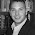## Wednesday, May 12, 2010

### Simple but profound applications of thermodynamics

Today I gave a lecture on the phase diagrams of carbon and superconductors.

I showed a couple of cool videos:

One student had a question I did not have a good answer to:

How does BCS theory explain that the Gibbs free energy of the superconducting state is lower than the metallic state?

1.How does BCS theory explain that the Gibbs free energy of the superconducting state is lower than the metallic state?

Here's my answer, I'd welcome questions or corrections:

i) Superconductivity is the low temperature phase so we know it will be have lower enthalpy, H, and lower entropy, S, than the normal metal (G=H-TS). So really we need to explain how come the enthalpy is lower.

ii) Basically the Cooper problem explains this. The electrons at the Fermi energy feel a next attractive force, so they can lower there enthalpy (or energy if you prefer to work at T=0) by forming singlets. These are the Cooper pairs, which one also finds in BCS theory.

In the full blown BCS theory one finds that the free energy is lower by ~|Delta}^2 - where Delta is the superconducting gap.

I think James Annett's book has a quite accessible discussion of this - but I haven't double checked.

2.The entropy should be larger for the metallic state, which is why the superconducting state is realized at low temperatures. As T-> 0 we have to consider more closely the enthalpy as it will dominate the free energy in the low-temperature regime. I assume that the enthalpy is lower for the superconducting state because of reduced Fermi pressure. The fermion statistics induce an effective pressure in the metallic state as the fermions are not capable of occupying the same state. The formation of Cooper pairs results in the now bosonic singlet pairs condensing into the lowest-energy state. This can be thought of as a reduced Fermion or (degeneracy) pressure. It's a purely quantum mechanical effect that is difficult to connect to a classical analogue (although since the enthalpy is pressure times volume, maybe you can make a tenuous connection).

3.The entropy is lower in the superconducting phase because the gap opens. In both the metal and the superconductor S=f(1-f), where f is the Fermi function. But, in the superconductor there are states near the Fermi energy that are gapped out, hence there is less entropy.